# How to Find the Nth Term of an Arithmetic Sequence

How to Find the Nth Term of an Arithmetic Sequence• The nth term is a rule that is used to find any term in a sequence.
• To find the nth term, find the difference between each term and write this number before the n.
• This sequence goes up in twos so we start by writing the 2n sequence.
• The 2n sequence is just the 2 times table and it also goes up in twos.
• We then find what we need to add on to this to make our original sequence.
• Comparing the first terms, we can see that we add 1 to 2 to make 3.
• If we add one to each number in the two times table, we get the terms of our sequence.
• We add one to the 2n sequence so the nth term is 2n + 1.

Find the difference between each term and write this number in front of n.

Find the difference between this number and the first term in the sequence and add this on.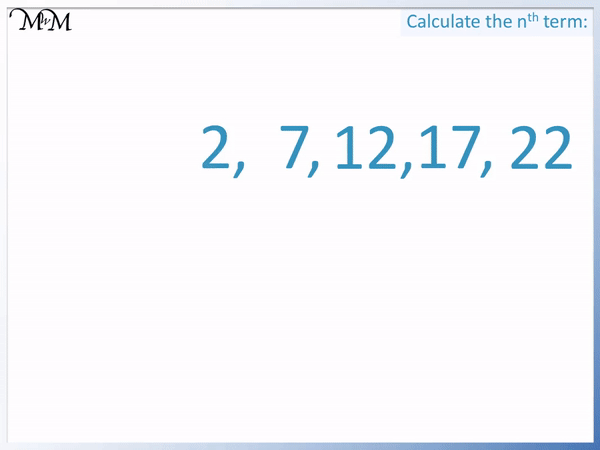• The terms in the sequence are going up by 5 each time.
• So we look at the 5n sequence.
• The 5n sequence is the five times table: 5, 10, 15, 20 etc.
• We can see that we subtract 3 from each term in the 5 times table to make our sequence.
• Therefore the sequence nth term is 5n – 3.Supporting Lessons# The Nth Term of an Arithmetic Sequence

## What is the Nth Term?

The nth term is a formula used to generate any term of a sequence. To find a given term, substitute the corresponding value of n into the nth term formula. For example, if the nth term is 3n + 2, the 10th term of the sequence can be found by substituting n = 10 into the nth term. 3 × 10 + 2 = 32 and so, the tenth term of the sequence is 32.The nth term can be used to generate a sequence. To generate a sequence using the nth term, substitute consecutive values of n into the sequence starting from n = 1.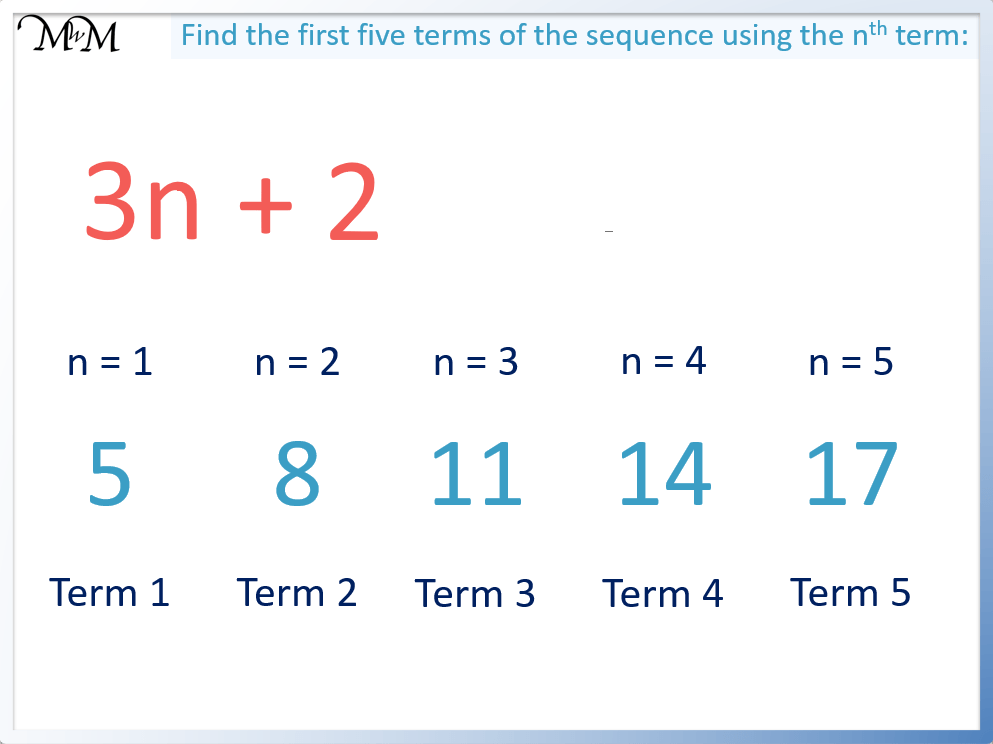Here is a list of calculations for generating the first 5 terms of the sequence 3n + 2.

• When n = 1, 3 × 1 + 2 = 5. The 1st term is 5.
• When n = 2, 3 × 2 + 2 = 8. The 2nd term is 8.
• When n = 3, 3 × 3 + 2 = 11. The 3rd term is 11.
• When n = 4, 3 × 4 + 2 = 14. The 4th term is 14.
• When n = 5, 3 × 5 + 2 = 17. The 5th term is 17.

Here is a table showing the first ten terms of the sequence 3n + 2 using the nth term formula.

nth Term Value of n Calculation Term
3n + 2 1 3 × 1 + 2 = 5
3n + 2 2 3 × 2 + 2 = 8
3n + 2 3 3 × 3 + 2 = 11
3n + 2 4 3 × 4 + 2 = 14
3n + 2 5 3 × 5 + 2 = 17
3n + 2 6 3 × 6 + 2 = 20
3n + 2 7 3 × 7 + 2 = 23
3n + 2 8 3 × 8 + 2 = 26
3n + 2 9 3 × 9 + 2 = 29
3n + 2 10 3 × 10 + 2 = 32

## What is an Arithmetic Sequence?

An arithmetic sequence is a list of numbers that always increase or decrease by the same amount from one number to the next. We say that in an arithmetic sequence, there is a common difference between the terms. For example, the sequence of 3, 5, 7, 9, 11… is arithmetic because the difference between each term is 2. We add 2 from one term to the next and so, the common difference is 2.The number in front of the n in the arithmetic sequence formula tells us what the difference is between each term in the sequence.

For example in the sequence above, the nth term is 2n + 1. The terms increase by 2 each time because there is a 2 in front of the n in the formula.

The most basic arithmetic sequences are the times tables. We can form sequences for the times tables by writing the appropriate number in front of n.

For example, the nth term for the 5 times table is just 5n.Substituting n = 1, n =2 etc into the 5n sequence we get 5, 10, 15, 20 and so on.We can see that the common difference between the terms in the 5 times table is 5. We add 5 to get from one number to the next.

The same can be seen in the 3 times table with the 3n sequence.Again, the 3 times table goes up in threes and is formed by writing a 3 in front of the n.

Arithmetic sequences can also decrease from one term to the next. For example, the arithmetic sequence formed by -2n + 10 starts at 8 and goes down by 2 each time. This is because the number in front of the n is -2.Decreasing arithmetic sequences always decrease by the same amount from one term to the next. The number in front of the n in the nth term for a decreasing arithmetic sequence tells us how much the terms decrease by each time. For example, with -2n + 10, the terms decrease by 2 each time.

## How to Find the Nth Term of an Arithmetic Sequence

To find the nth term of an arithmetic sequence, find the common difference between each term. Start by writing this difference multiplied by n. Then list multiples of the difference and see what needs to be added or subtracted to this to make the original sequence. For example 3, 7, 11, 15, 19… increase by 4 each time so we start with the 4n sequence: 4, 8, 12, 16, 20. We can see that we subtract 1 from each term in the 4n sequence to make the original sequence and so, the nth term is 4n – 1.The number in front of the n in the nth term formula tells us how much we are increasing or decreasing by.

Since the terms in this example increase by 4, we write a 4 in front of the n.

The 4n sequence is the four times table. We compare each term in the 4 times table to see what we need to adjust it by to make our sequence. It helps to list the two sequences above each other to compare them.

If we subtract 1 from the 4n sequence, we get the numbers in our original sequence. For example, 4 – 1 = 3, 8 – 1 = 7 and so on.

The nth term is 4n – 1.

## How to Easily Find the Nth Term of a Sequence

To find the nth term of a sequence, use these steps:

1. Find the common difference from one term to the next.
2. Write this difference with an ‘n’ after it.
3. Subtract the difference from the first term in the sequence.

For example, we will find the nth term for the sequence starting 3, 7, 11, 15, 19…

Step 1. Find the common difference: The terms increase by 4 each time.

Step 2. Write this difference with an ‘n’ after it: ‘4n’.

Step 3. Subtract the difference from the 1st term: 3 – 4 = -1.

Step 4. Write this after the answer to step 2: ‘4n – 1’.

And so the nth term of our sequence is 4n – 1.## Nth Term of a Decreasing Sequence

If a sequence is decreasing from one term to the next, the number in front of n in the nth term will have a negative sign. For example, in the sequence 8, 6, 4, 2, 0… the terms decrease by 2 so we start by writing -2n. The -2n sequence is -2, -4, -6, -8, -10 and so on. We need to add 10 to each of these terms to make the original sequence, so the nth term is -2n + 10.The -2n sequence is simply the negative 2 times table.

We need to add 10 to each of these terms to make the sequence 8, 6, 4, 2, 0…

For instance, -2 + 10 = 8, -4 + 10 = 6 and so on.

Here is another example of finding the nth term of a decreasing sequence. We have -5, -8, -11, -14, -17…

The sequence decreases by 3 from one term to the next. Therefore the common difference is -3.

We start by looking at the -3n sequence, which is just the negative 3 times table: -3, -6, -9, -12, -15…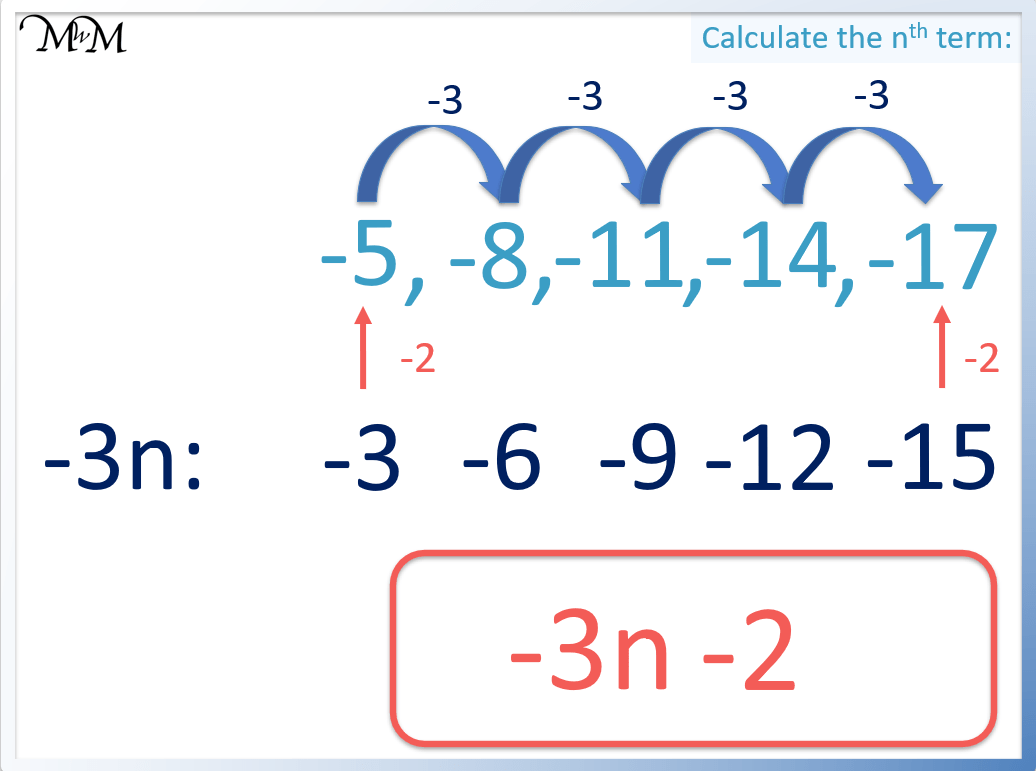We need to take away 2 more from the -3n sequence to make our original sequence. For example, -3 – 2 = -5 and -6 – 2 = -8.

Therefore the nth term is -3n – 2.

## How to Find the Nth Term of a Sequence with Decimals

The rule for finding the nth term of a sequence still applies to decimal numbers. The difference between each term is written in front of the n and the adjustment that needs to be added is written at the end.

For example, find the nth term for the decimal sequence 0.3, 0.4, 0.5, 0.6…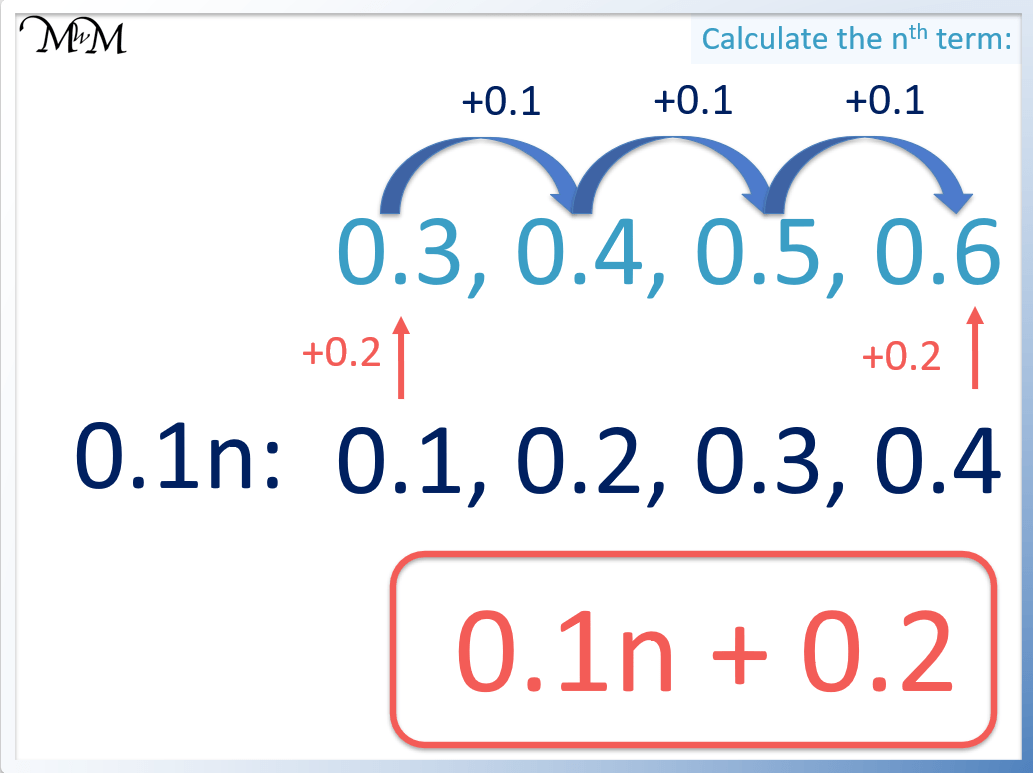The difference from one term to the next is 0.1. Therefore we start with 0.1n.

The 0.1n sequence is 0.1, 0.2, 0.3 and so on.

We need to add 0.2 to each of these terms to make our sequence of 0.3, 0.5, 0.6 and so on.

Therefore the nth term of this decimal sequence is 0.1n + 0.2.

Here is another example of finding the nth term of a decimal sequence. We have 1.2, 1.5, 1.8, 2.1 and so on.The term-to-term difference is 0.3. We add 0.3 from one term to the next.

So we start with the 0.3n sequence: 0.3, 0.6, 0.9 and so on.

We must add 0.9 to the 0.3n sequence to make the original sequence of 1.2, 1.5, 1.8 and so on.

Therefore the nth term of this decimal sequence is 0.3n + 0.9.

## Nth Term of an Arithmetic Sequence Examples

Here are several examples of finding the nth term of an arithmetic sequence.

Sequence Difference Multiples of the Difference What do we add to this? Nth Term
3, 5, 7, 9, 11 … +2 2n sequence: 2, 4, 6, 8, 10 +1 2n + 1
10, 13, 16, 19, 22 … +3 3n sequence: 3, 6, 9, 12, 15 +7 3n + 7
1, 6, 11, 16, 21 … +5 5n sequence: 5, 10, 15, 20, 25 -4 5n – 4
4, 14, 24, 34, 44 … +10 10n sequence: 10, 20, 30, 40, 50 -6 10n – 6
20, 17, 14, 11, 8 … -3 -3n sequence: -3, -6, -9, -12, -15 +23 -3n + 23
-6, -10, -14, -18, -22 … -4 -4n sequence: -4, -8, -12, -16, -20 -2 -4n – 2

## Formula for the Nth Term of an Arithmetic Sequence

The formula to find the nth term of an arithmetic sequence is an = a1 + (n-1)d, where an is the nth term, a1 is the 1st term, n is the term number and d is the common difference. To find the formula for the nth term, we need a1 and d. For example, in the sequence 5, 7, 9, 11, 13… a1 = 5 and d = 2. an = a1 + (n-1)d becomes an = 5 + 2(n-1), which simplifies to an = 2n + 3.To find the formula for the nth term of an arithmetic sequence, we need to know the difference, ‘d’, and the first term, ‘a1‘.

In this example, the first term is 5 and the common difference is 2.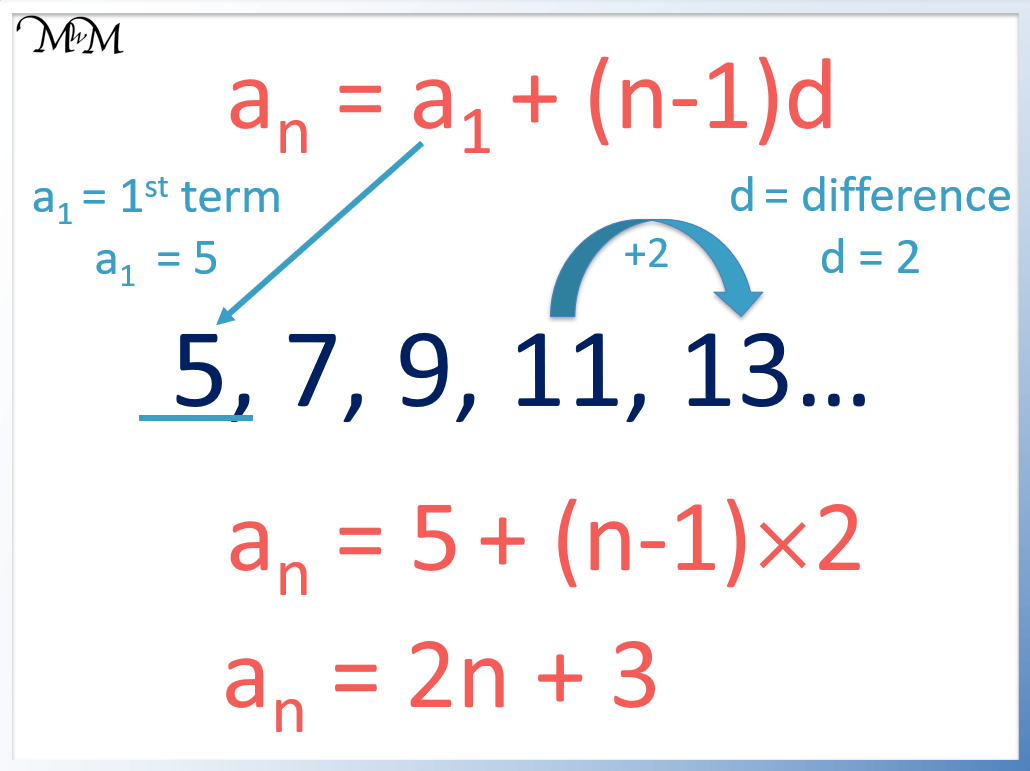Substituting the values of a1= 5 and d = 2 into an = a1 + (n-1)d, we get an = 5 + (n-1)2.

We simplify this by first expanding the brackets by multiplying (n-1) by 2.

We get an = 5 + 2n – 2. This simplifies to an = 2n + 3.Now try our lesson on Negative Numbers on a Number Line where we learn about negative numbers.error: Content is protected !!